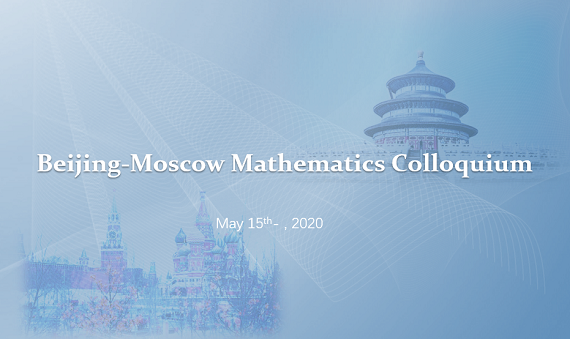## Joint Moscow-Beijing Mathematical Online Colloquium «Beijing-Moscow Mathematics Colloquium»##### 11:00 (Moscow time)
 Prof. A.S. Mishchenko Lomonosov Moscow State University Bio: Professor A.S. Mischenko graduated from Moscow State University in 1965. He became a Professor of the Department of Higher Geometry and Topology, Faculty of Mechanics and Mathematics of this University in 1979. He also holds a position of Leading researcher at the Mathematical Steklov Institute. He is a Honored Professor of Moscow University since 2006. His research interests include geometry and topology and their applications. The main direction of his work is related to the study and application of algebraic and functional methods in the theory of smooth manifolds.

### Geometric description of the Hochschild cohomology of Group Algebras

There are two approaches to the study of the cohomology of group algebras ℝ[G]: the Eilenberg-MacLane cohomology and the Hochschild cohomology. In the case of Eilenberg-MacLane cohomology one has the classical cohomology of the classifying space BG. Hochschild cohomology represents a more general construction, in which so-called two-sided bimodules are considered.

The Hochschild cohomology and the usual Eilenberg-MacLane cohomology are coordinated by moving from bimodules to left modules.

For the Eilenberg-MacLane cohomology, in the case of a nontrivial action of the group G in the module Ml , no reasonable geometric interpretation has been known so far. An effective geometric description of the Hochschild cohomology is devoted to the main result of this paper. The key point for the new geometric description of the Hochschild cohomology is the new groupoid Gr associated with the adjoint action of the group G.

The cohomology of the classifying space BGr of this groupoid with an appropriate condition for the finiteness of the support of cochains is isomorphic to the Hochschild cohomology of the algebra ℝ[G]. Hochschild homology is described in the form of homology groups of the space BGr, but without any conditions of finiteness on chains.

There is a connection between the homology of the space BGr and the cohomology of BGr in the form of an isomorphism H*f(BGr, ℝ) ≈ Homf(H*(BGr), ℝ) where Homf is a set of linear homomorphisms with a condition of finiteness.

##### 12:00 (Moscow time)
 Prof. Yu Jun Beijing International Center for Mathematical Research Bio: Jun Yu obtained PhD from ETH Zurich in 2012, and then did postdoc in IAS Princeton and MIT. He is now an assistant professor in Beijing international center for mathematical research at Peking University. His research field is representation theory and Langlands program, including the branching rule problem, the orbit method philosophy, and the beyond endoscopy program.

### Restriction of unitary representations of Spin(N,1) to parabolic subgroups

The orbit method predicts a relation between restrictions of irreducible unitary representations and projections of corresponding coadjoint orbits. In this talk we will discuss branching laws for unitary representations of Spin(N,1) restricted to parabolic subgroups and the corresponding orbit geometry. In particular, we confirm Duflo's conjecture in this setting. This is a joint work with Gang Liu (Lorraine) and Yoshiki Oshima (Osaka).

The meeting will be held in the form of a webinar on the Zoom platform.

Pre-registration for the event is not required.

To join Zoom meeting（You can join in the meeting without a phone number）:

Zoom ID：650 2161 708